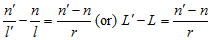# fundamental paraxial equation

Also found in: Encyclopedia.

## paraxial equation, fundamental

Equation based on gaussian theory and dealing with refraction at a spherical surface:where n and n′ are the refractive indices of the media on each side of the spherical surface, r is the radius of curvature of the surface and l and l′ the distances of the object and the image from the surface, respectively. n/l and n′ /l′ are the vergences (or reduced vergences) of the incident and refracted light rays respectively. L′ − L corresponds to the change produced by the surface in the vergence of the light and is called the focal power (or vergence power, or refractive power) F of the surface. Thus
L′ − L = F
Focal power is usually expressed in dioptres and can be either positive or negative.At a reflecting surface or a mirror the equation becomes
L′ − L = 2/r
where r is the radius of curvature of the surface or mirror. Syn. general refraction formula. See image distance; object distance; sign convention; gaussian theory; vergence.
Millodot: Dictionary of Optometry and Visual Science, 7th edition. © 2009 Butterworth-Heinemann
Site: Follow: Share:
Open / Close